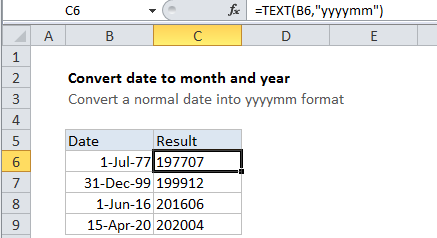## Excel Office

Excel How Tos, Tutorials, Tips & Tricks, Shortcuts

# Convert date to month and year in Excel

This tutorial shows how to Convert date to month and year in Excel using example below.

To convert a normal Excel date into yyyymm format (e.g. 9/1/2017 > 201709), you can use the TEXT function.

## Formula

`=TEXT(date,"yyyymm")`## Explanation

In the example shown, the formula in C6 is:

`=TEXT(B6,"yyyymm")`

### How this formula works

The TEXT function applies the number format you specify to a numeric value, and returns a result as text.

Also See:   Get days, hours, and minutes between dates in Excel

In this case, the number format provided is “yyyymm”, which joins a 4-digit year with a 2-digit month value.

### Display only option

If you only want to display a date with the year and month, you can simply apply the custom number format “yyyymm” to the date(s). This will cause Excel to display the year and month together, but will not change the underlying date.

Also See:   How to show last updated date stamp in Excel

1.